Скачать презентацию Semiparametric Methods for Colonic Crypt Signaling Raymond J

• Количество слайдов: 35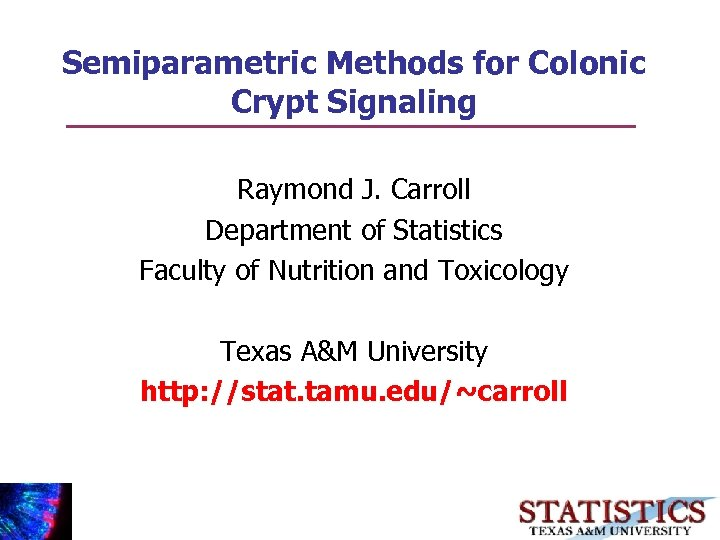Semiparametric Methods for Colonic Crypt Signaling Raymond J. Carroll Department of Statistics Faculty of Nutrition and Toxicology Texas A&M University http: //stat. tamu. edu/~carroll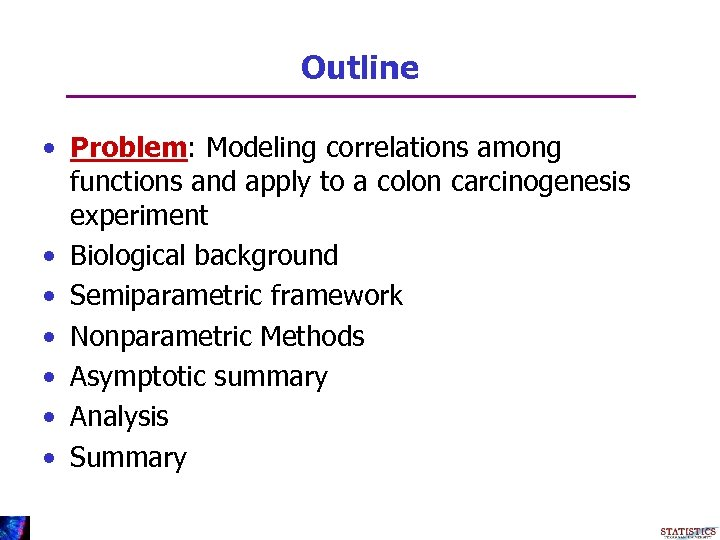Outline • Problem: Modeling correlations among functions and apply to a colon carcinogenesis experiment • Biological background • Semiparametric framework • Nonparametric Methods • Asymptotic summary • Analysis • Summary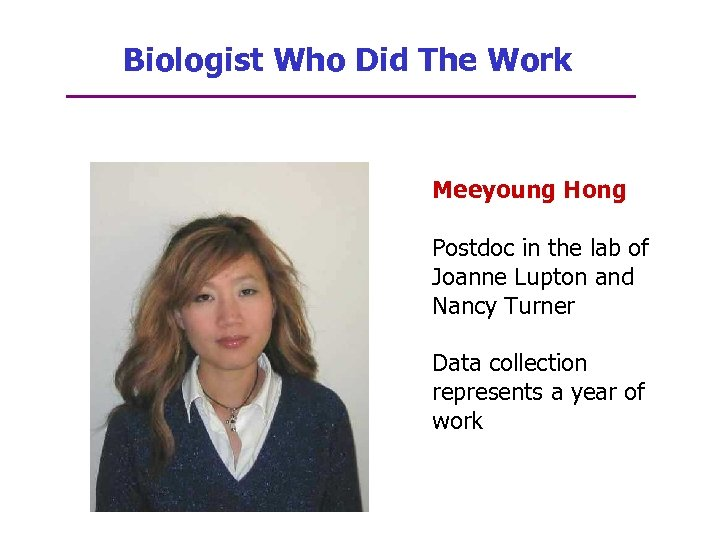Biologist Who Did The Work Meeyoung Hong Postdoc in the lab of Joanne Lupton and Nancy Turner Data collection represents a year of work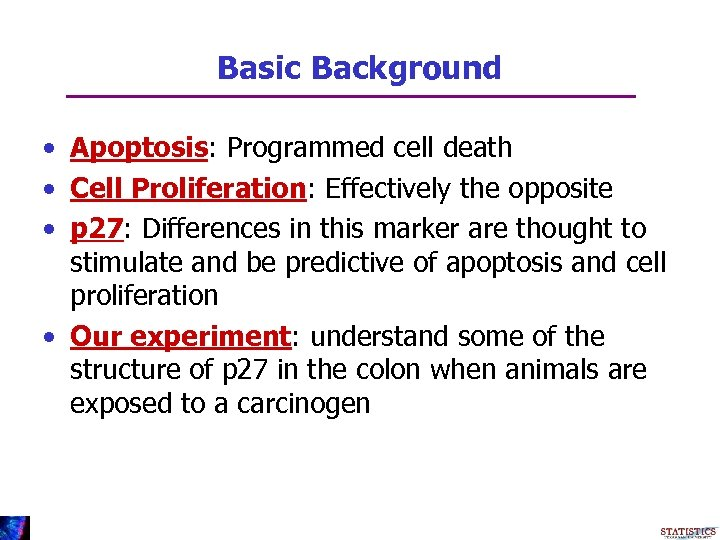Basic Background • Apoptosis: Programmed cell death • Cell Proliferation: Effectively the opposite • p 27: Differences in this marker are thought to stimulate and be predictive of apoptosis and cell proliferation • Our experiment: understand some of the structure of p 27 in the colon when animals are exposed to a carcinogen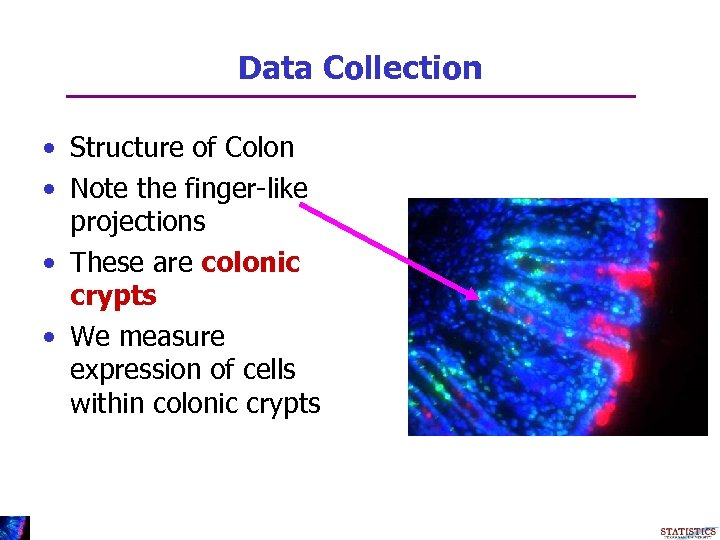Data Collection • Structure of Colon • Note the finger-like projections • These are colonic crypts • We measure expression of cells within colonic crypts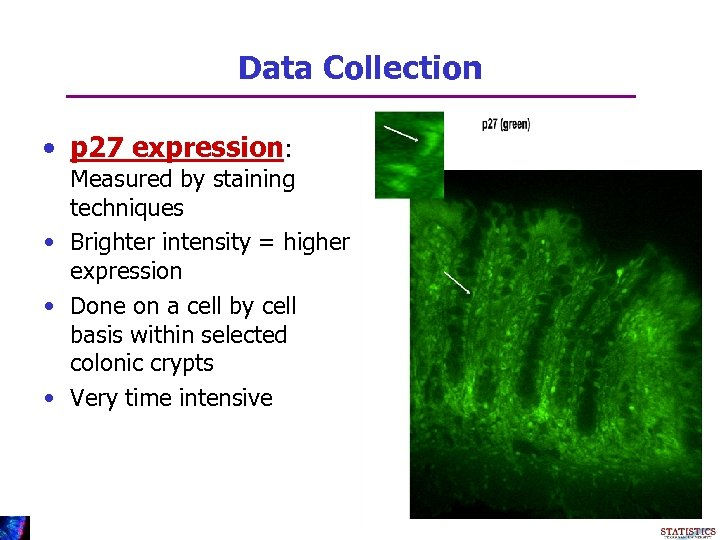Data Collection • p 27 expression: Measured by staining techniques • Brighter intensity = higher expression • Done on a cell by cell basis within selected colonic crypts • Very time intensive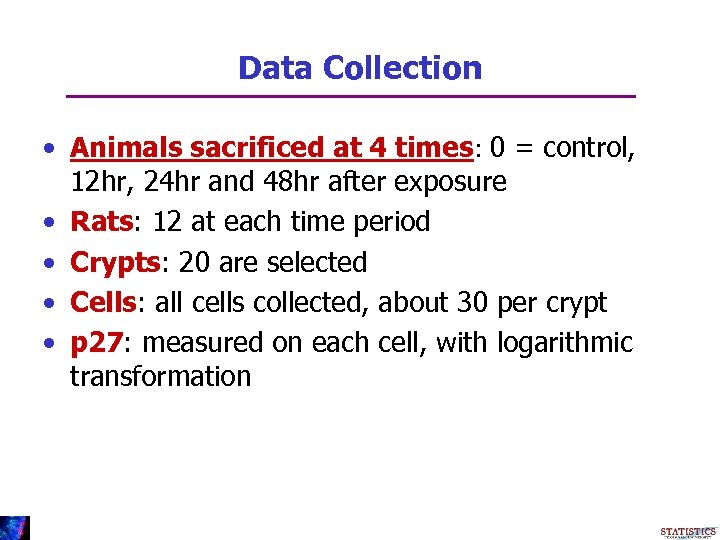Data Collection • Animals sacrificed at 4 times: 0 = control, 12 hr, 24 hr and 48 hr after exposure • Rats: 12 at each time period • Crypts: 20 are selected • Cells: all cells collected, about 30 per crypt • p 27: measured on each cell, with logarithmic transformation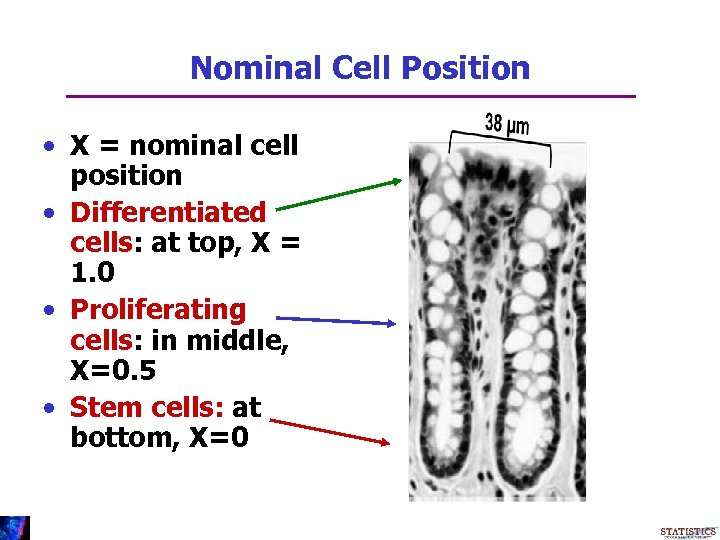Nominal Cell Position • X = nominal cell position • Differentiated cells: at top, X = 1. 0 • Proliferating cells: in middle, X=0. 5 • Stem cells: at bottom, X=0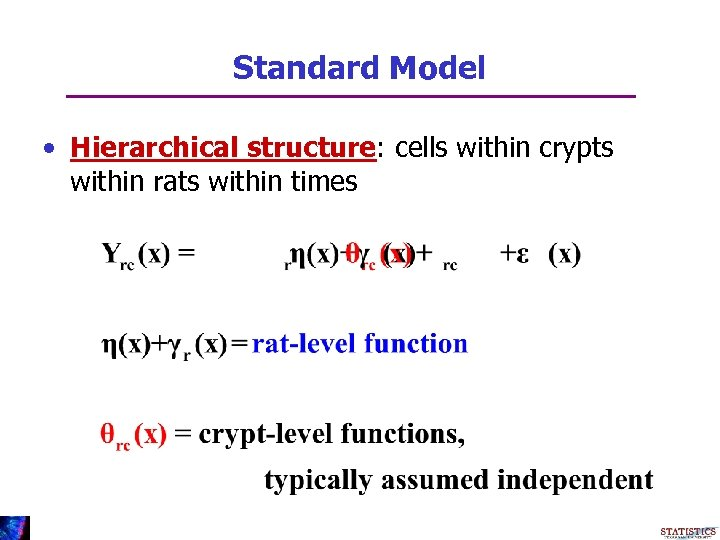Standard Model • Hierarchical structure: cells within crypts within rats within times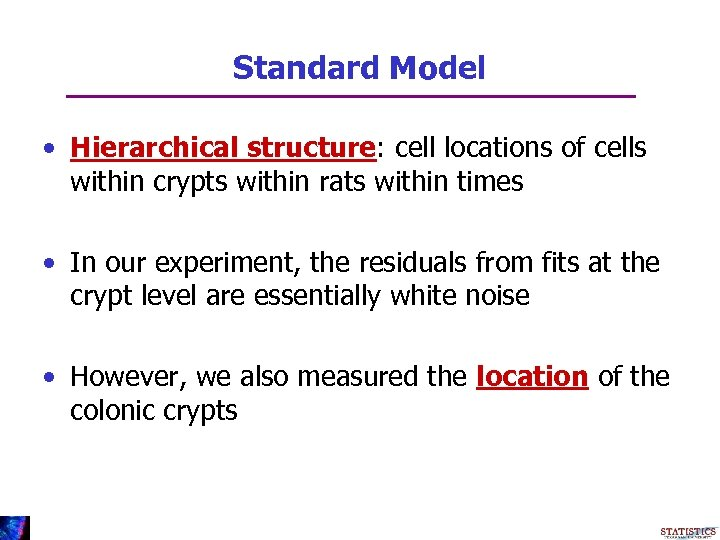Standard Model • Hierarchical structure: cell locations of cells within crypts within rats within times • In our experiment, the residuals from fits at the crypt level are essentially white noise • However, we also measured the location of the colonic crypts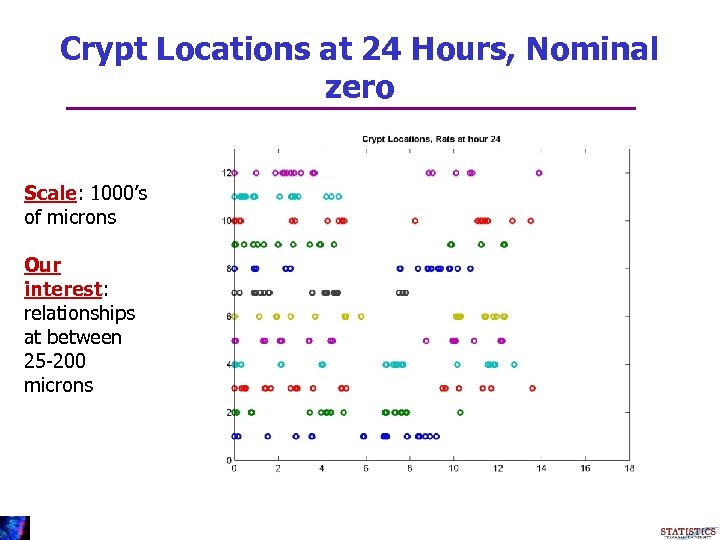Crypt Locations at 24 Hours, Nominal zero Scale: 1000’s of microns Our interest: relationships at between 25 -200 microns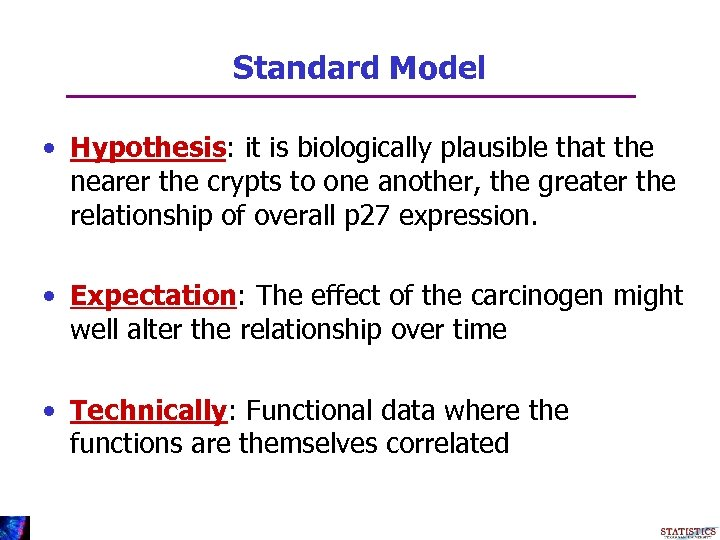Standard Model • Hypothesis: it is biologically plausible that the nearer the crypts to one another, the greater the relationship of overall p 27 expression. • Expectation: The effect of the carcinogen might well alter the relationship over time • Technically: Functional data where the functions are themselves correlated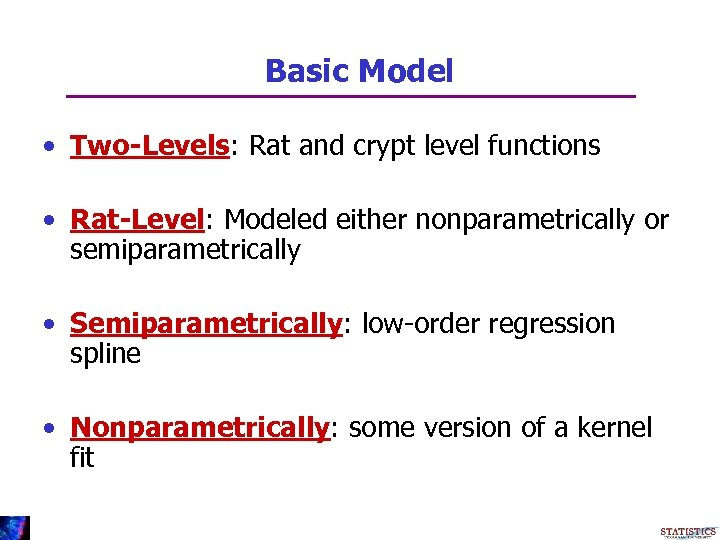Basic Model • Two-Levels: Rat and crypt level functions • Rat-Level: Modeled either nonparametrically or semiparametrically • Semiparametrically: low-order regression spline • Nonparametrically: some version of a kernel fit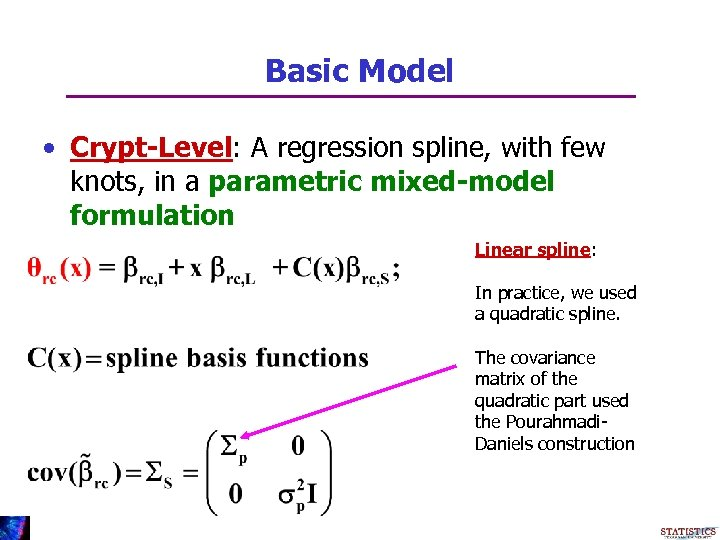Basic Model • Crypt-Level: A regression spline, with few knots, in a parametric mixed-model formulation Linear spline: In practice, we used a quadratic spline. The covariance matrix of the quadratic part used the Pourahmadi. Daniels construction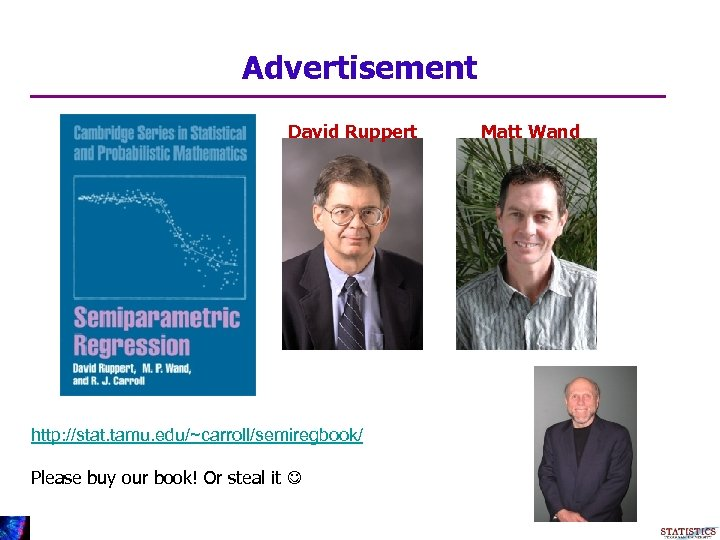Advertisement David Ruppert http: //stat. tamu. edu/~carroll/semiregbook/ Please buy our book! Or steal it Matt Wand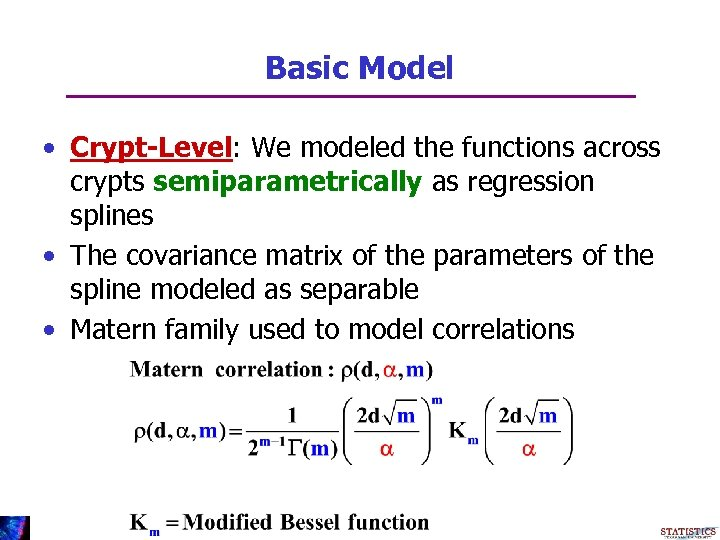Basic Model • Crypt-Level: We modeled the functions across crypts semiparametrically as regression splines • The covariance matrix of the parameters of the spline modeled as separable • Matern family used to model correlations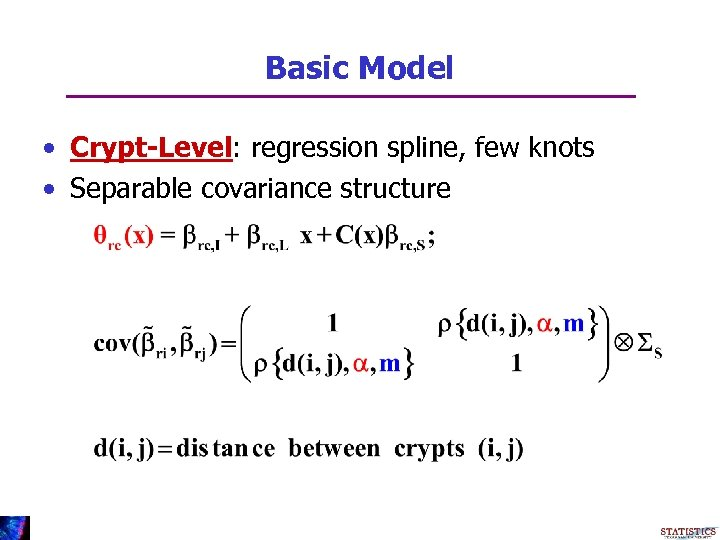Basic Model • Crypt-Level: regression spline, few knots • Separable covariance structure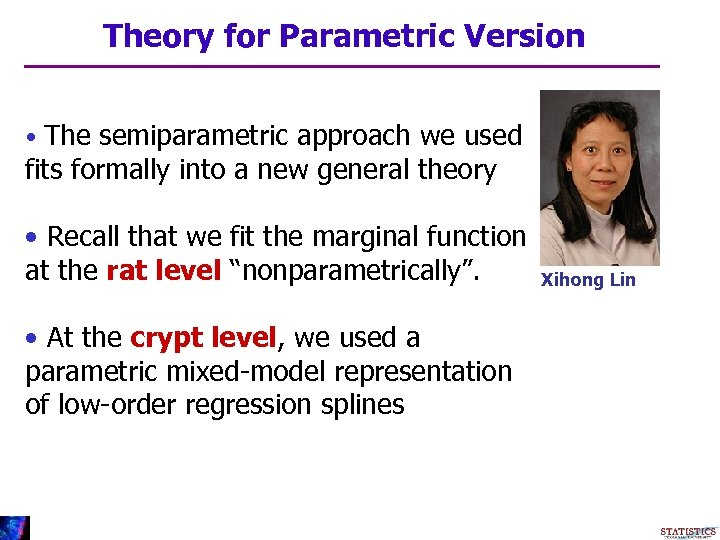Theory for Parametric Version • The semiparametric approach we used fits formally into a new general theory • Recall that we fit the marginal function at the rat level “nonparametrically”. • At the crypt level, we used a parametric mixed-model representation of low-order regression splines Xihong Lin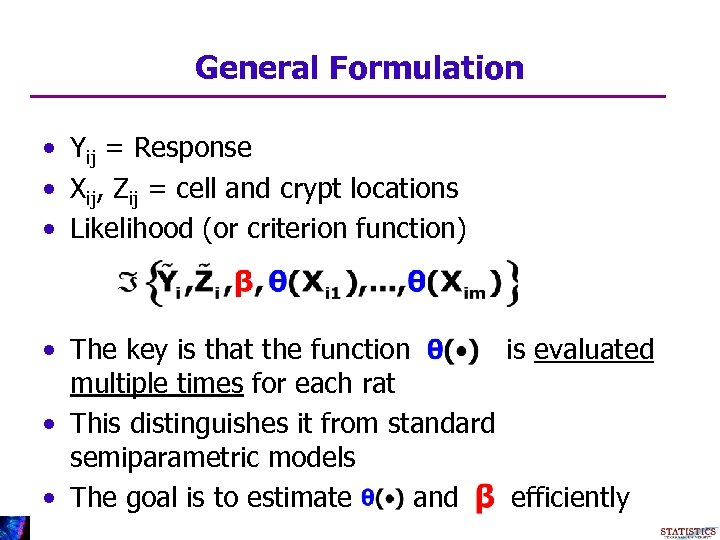General Formulation • Yij = Response • Xij, Zij = cell and crypt locations • Likelihood (or criterion function) • The key is that the function is evaluated multiple times for each rat • This distinguishes it from standard semiparametric models • The goal is to estimate and efficiently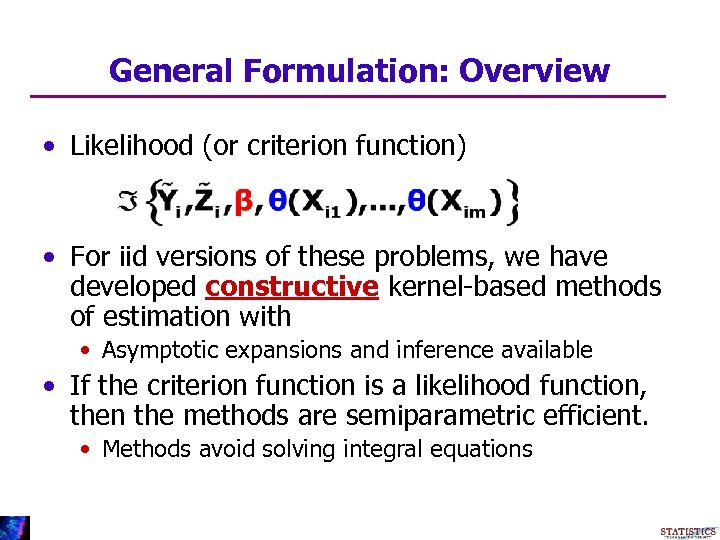General Formulation: Overview • Likelihood (or criterion function) • For iid versions of these problems, we have developed constructive kernel-based methods of estimation with • Asymptotic expansions and inference available • If the criterion function is a likelihood function, then the methods are semiparametric efficient. • Methods avoid solving integral equations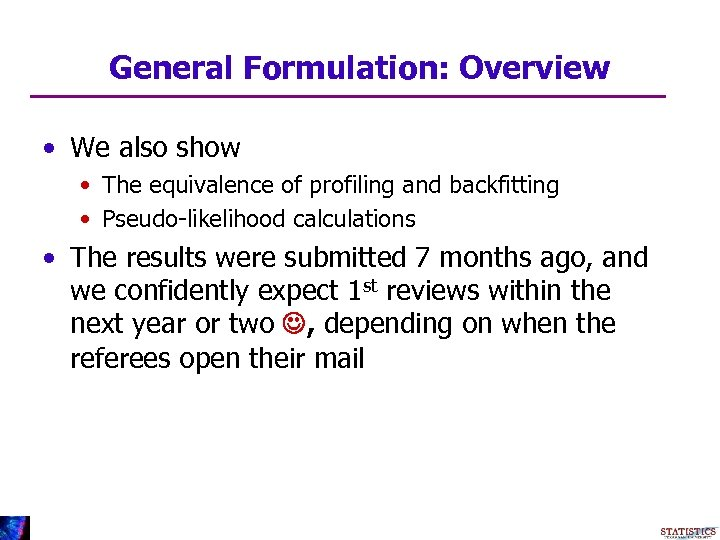General Formulation: Overview • We also show • The equivalence of profiling and backfitting • Pseudo-likelihood calculations • The results were submitted 7 months ago, and we confidently expect 1 st reviews within the next year or two , depending on when the referees open their mail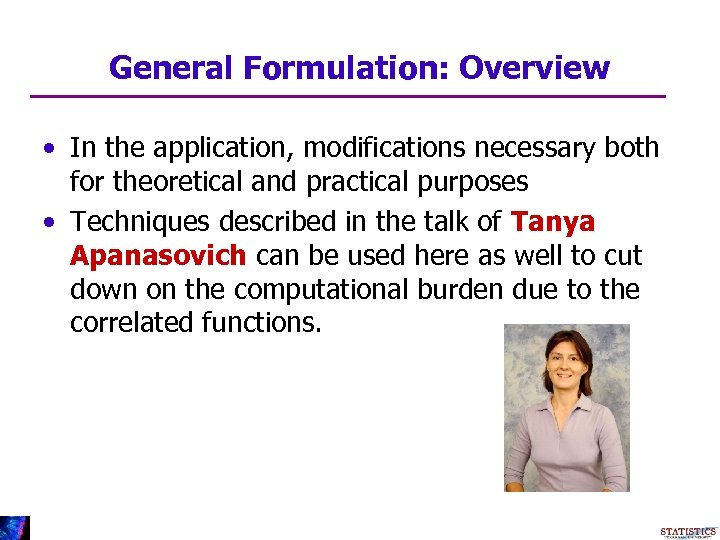General Formulation: Overview • In the application, modifications necessary both for theoretical and practical purposes • Techniques described in the talk of Tanya Apanasovich can be used here as well to cut down on the computational burden due to the correlated functions.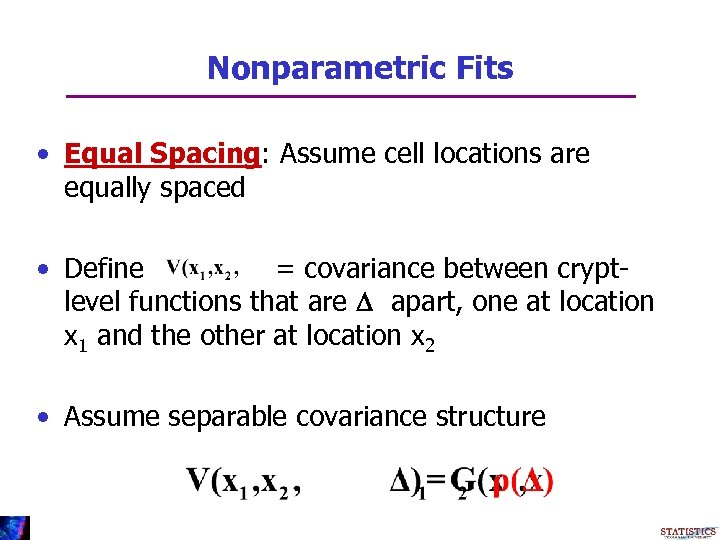Nonparametric Fits • Equal Spacing: Assume cell locations are equally spaced • Define = covariance between cryptlevel functions that are D apart, one at location x 1 and the other at location x 2 • Assume separable covariance structure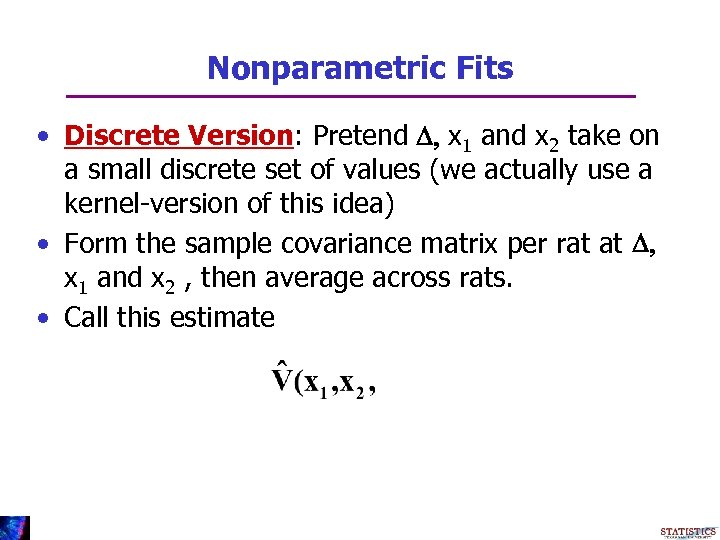Nonparametric Fits • Discrete Version: Pretend D, x 1 and x 2 take on a small discrete set of values (we actually use a kernel-version of this idea) • Form the sample covariance matrix per rat at D, x 1 and x 2 , then average across rats. • Call this estimate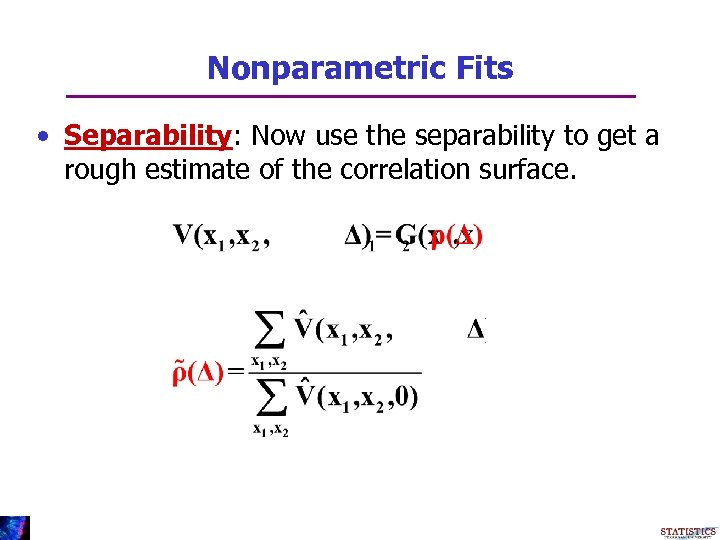Nonparametric Fits • Separability: Now use the separability to get a rough estimate of the correlation surface.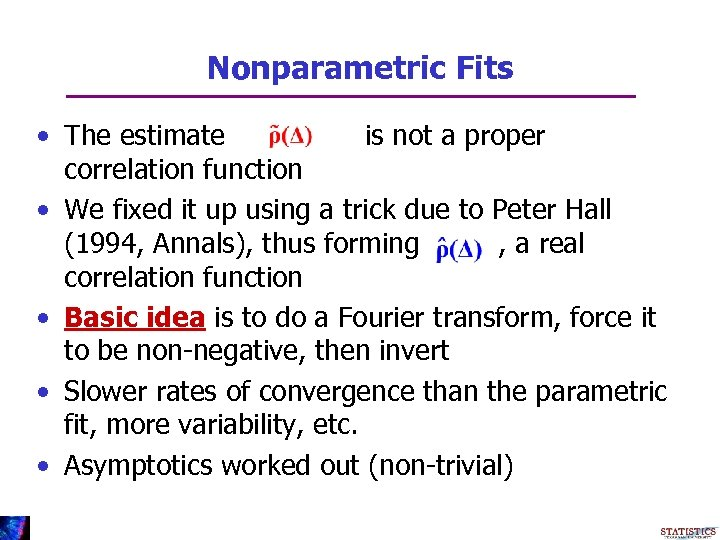Nonparametric Fits • The estimate is not a proper correlation function • We fixed it up using a trick due to Peter Hall (1994, Annals), thus forming , a real correlation function • Basic idea is to do a Fourier transform, force it to be non-negative, then invert • Slower rates of convergence than the parametric fit, more variability, etc. • Asymptotics worked out (non-trivial)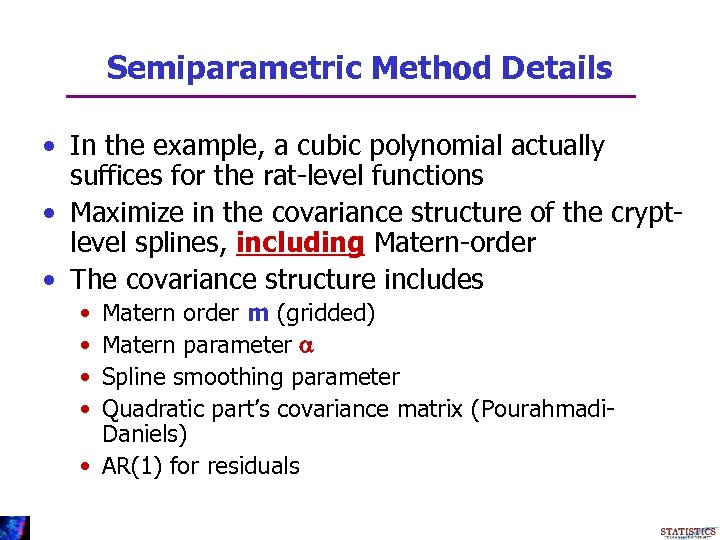Semiparametric Method Details • In the example, a cubic polynomial actually suffices for the rat-level functions • Maximize in the covariance structure of the cryptlevel splines, including Matern-order • The covariance structure includes • • Matern order m (gridded) Matern parameter a Spline smoothing parameter Quadratic part’s covariance matrix (Pourahmadi. Daniels) • AR(1) for residuals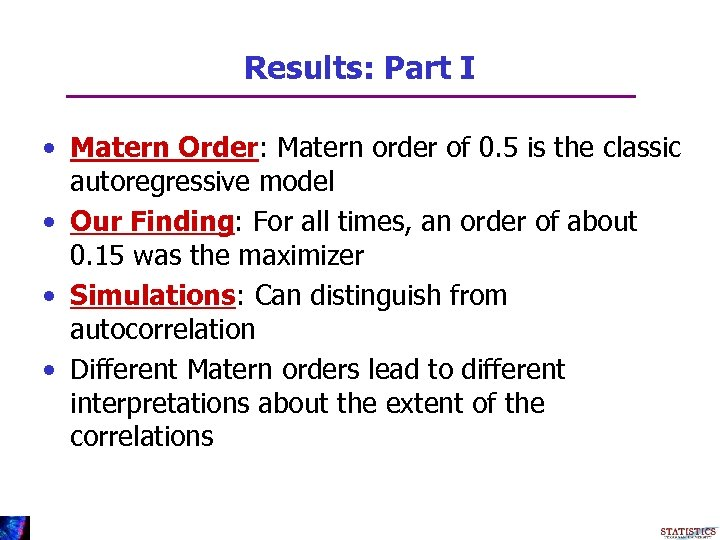Results: Part I • Matern Order: Matern order of 0. 5 is the classic autoregressive model • Our Finding: For all times, an order of about 0. 15 was the maximizer • Simulations: Can distinguish from autocorrelation • Different Matern orders lead to different interpretations about the extent of the correlations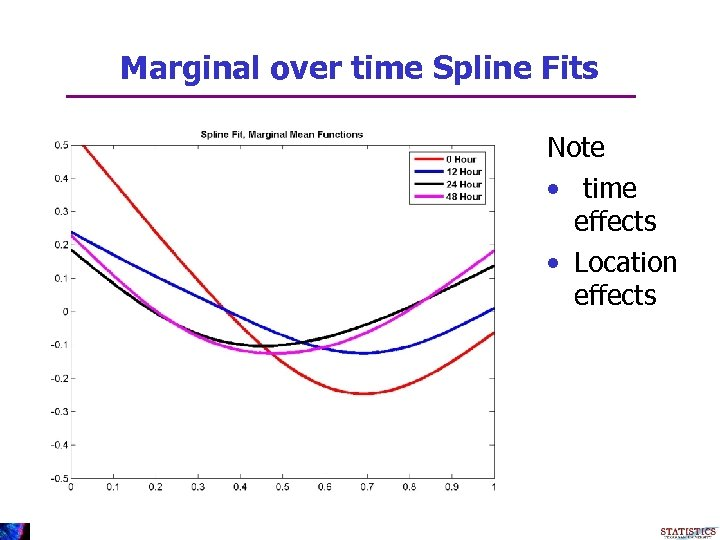Marginal over time Spline Fits Note • time effects • Location effects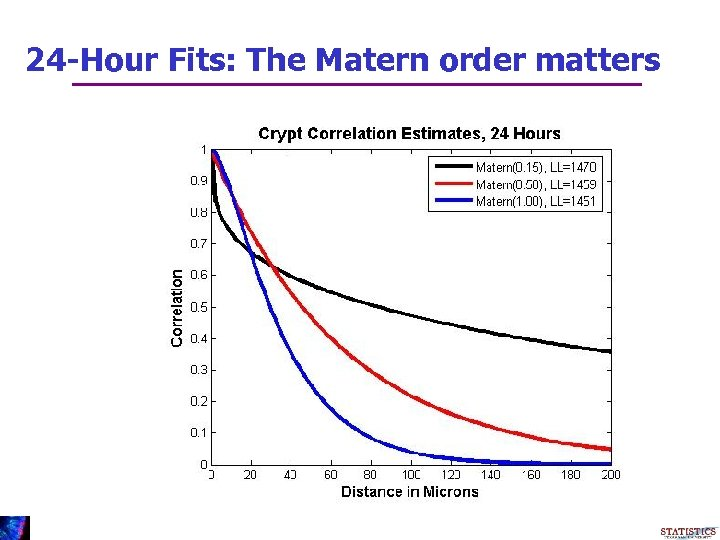24 -Hour Fits: The Matern order matters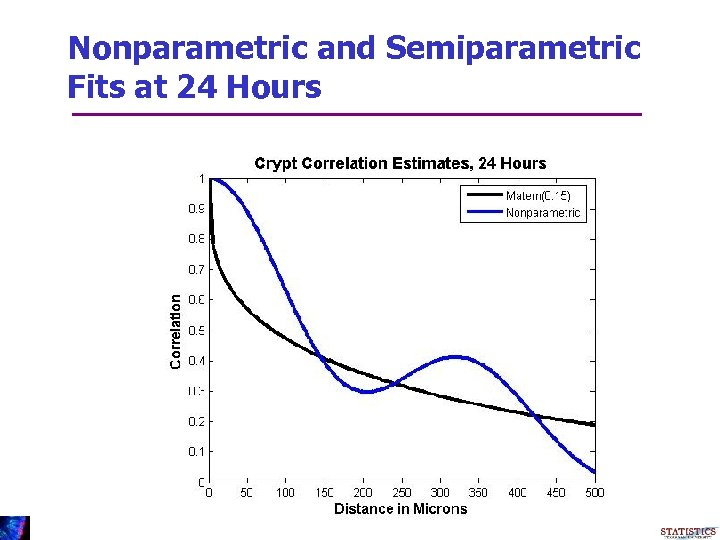Nonparametric and Semiparametric Fits at 24 Hours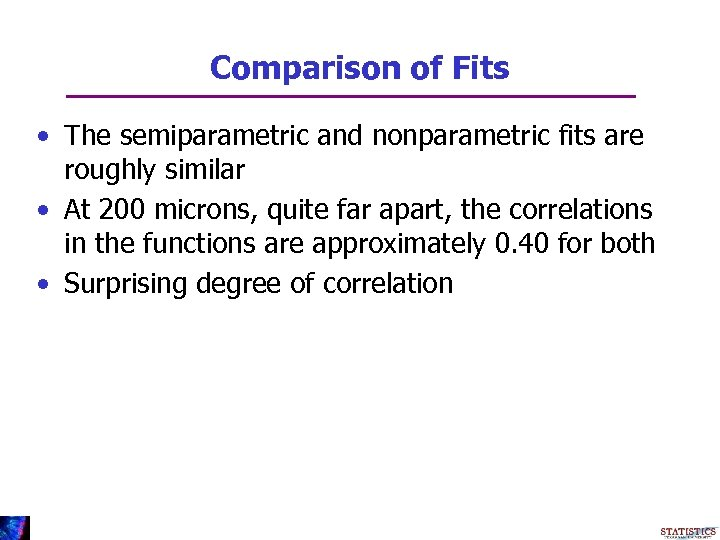Comparison of Fits • The semiparametric and nonparametric fits are roughly similar • At 200 microns, quite far apart, the correlations in the functions are approximately 0. 40 for both • Surprising degree of correlation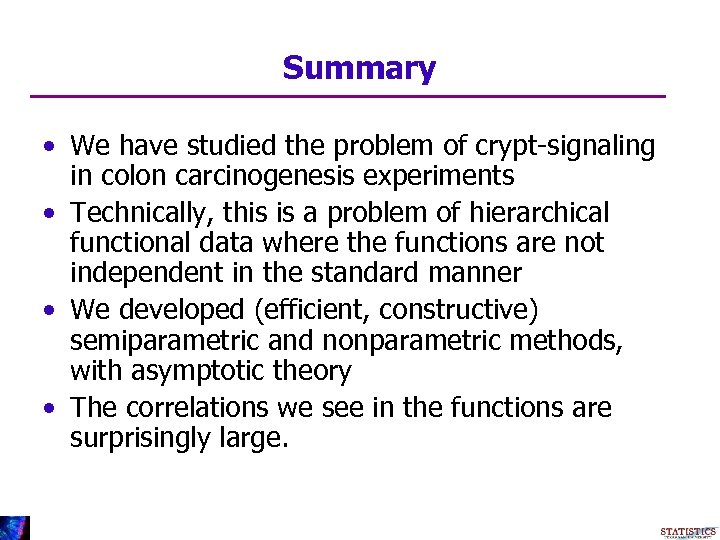Summary • We have studied the problem of crypt-signaling in colon carcinogenesis experiments • Technically, this is a problem of hierarchical functional data where the functions are not independent in the standard manner • We developed (efficient, constructive) semiparametric and nonparametric methods, with asymptotic theory • The correlations we see in the functions are surprisingly large.Statistical Collaborators Yehua Li Naisyin Wang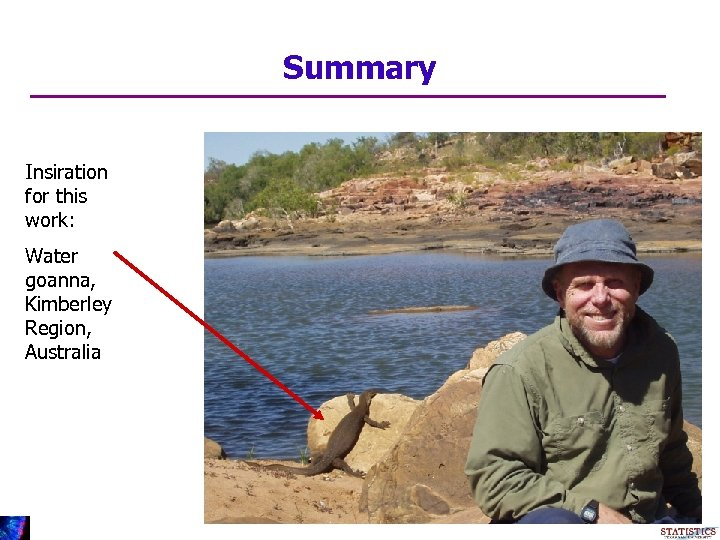Summary Insiration for this work: Water goanna, Kimberley Region, Australia